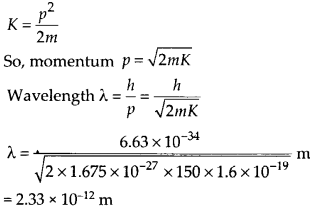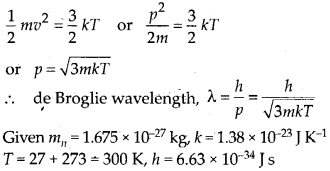Enlightened

# Question 32: NCERT Solutions for 12th Class Physics: Chapter 11-Dual Nature of Radiation and Matter

• 0

Question 32: NCERT Solutions for 12th Class Physics: Chapter 11-Dual Nature of Radiation and Matter

(a) Obtain the de Broglie wavelength of a neutron of kinetic energy 150 eV. An electron beam of this energy is suitable for crystal diffraction experiments. Would a neutron beam of the same energy be equally suitable? Explain. (mn = 1.675 × 10-27 kg)
(b) Obtain the de Broglie wavelength associated with thermal neutrons at room temperature (27°C). Hence explain why a fast neutron beam needs to be thermalised with the environment before it can be used for neutron diffraction experiments.

Share

1. Solution:
(a) Let us first calculate the wavelength of matter wave associated with neutron of kinetic energy 150 eV.As the inter atomic spacing (1 A= 10-10 m) is about hundred times greater than this wavelength, so a neutron beam of 150 eV energy is not suitable for diffraction experiments.

(b) Average kinetic energy of a neutron at absolute temperature T isAs this wavelength is comparable to inter atomic spacing (= 1 A) in a crystal, so thermal neutrons can be used for diffraction experiments. So high energy neutron beam should be first thermalised before using it for diffraction.Check the complete chapter with solutions.
NCERT Solutions for 12th Class Physics: Chapter 11-Dual Nature of Radiation and Matter

• 0